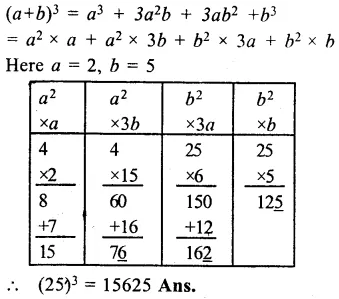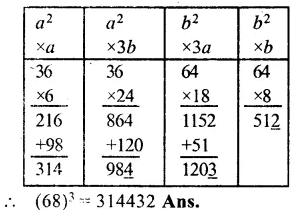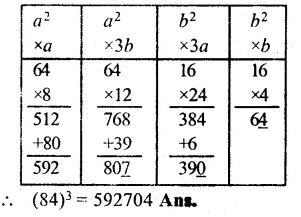## RS Aggarwal Class 8 Solutions Chapter 4 Cubes and Cube Roots Ex 4B

These Solutions are part of RS Aggarwal Solutions Class 8. Here we have given RS Aggarwal Solutions Class 8 Chapter 4 Cubes and Cube Roots Ex 4B.

Other Exercises

Find the value of each of the following using the short-cut method :

Question 1.
Solution:
(25)³ = We know that short-cut method for finding the cube of any two digit number is as given.Question 2.
Solution:
(47)³ = Here a = 4, b = 7Question 3.
Solution:
(68)³ = Here a = 6, b = 8Question 4.
Solution:
(84)³ Here a = 8, b = 4Hope given RS Aggarwal Solutions Class 8 Chapter 4 Cubes and Cube Roots Ex 4B are helpful to complete your math homework.

If you have any doubts, please comment below. Learn Insta try to provide online math tutoring for you.